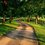# [Differential Geometry] Are Rotations on a Surface of Revolution Isometries?

Let S be a surface of revolution in $\mathbb{R}^3$. Are the rotations about its axis all isometries of S?

Without loss of generality, let's assume that the axis of revolution is the z-axis, and the generating curve in the xz-plane is parametrised by a unit speed parametrised curve $\alpha: I \rightarrow \mathbb{R}^3$, where $u \in I$ is $\alpha(u) = (f(u), 0, g(u)).$ Then by rotating this planar curve in the z-axis, we have the parametrisation

$\varphi(u,v) = (f(u)\cos v, f(u) \sin v, g(u))$ of $S$.

The coefficients of the first fundamental form of this parametrisation are $E=1, F=0, G= f^2(u).$ Since we let the generating curve be parametrised by unit speed curve, $\varphi$ does not depend on $g$. We can express the rotations by angle $\theta$ about the z-axis in the standard basis of $\mathbb{R}^3$:

$R_z(\theta) = \begin{bmatrix} \cos\theta & -\sin\theta & 0 \\ \sin\theta & \cos\theta & 0 \\ 0 & 0 & 1 \end{bmatrix}$

By composing this rotation with our parametrisation, we have $\varphi_{\theta}(u,v) = (R_z(\theta)\circ \varphi)(u,v) = (f(u)\cos(v+\theta),~ f(u)\sin(v+\theta),~ g(u)).$

Hence the coefficients of the first fundamental form of the surface after rotation, $\varphi_{\theta}$ are

$E_{\theta} = \left<\varphi_{\theta u}, \varphi_{\theta u}\right> = (f'(u))^2(\cos^2(v+\theta) + \sin^2(v+\theta))+(g'(u))^2 = (f'(u))^2+(g'(u))^2 = 1$ $F_{\theta} = \left<\varphi_{\theta u}, \varphi_{\theta v}\right> = -(f'(u))^2\cos(v+\theta)\sin(v+\theta) +(f'(u))^2\cos(v+\theta)\sin(v+\theta) + 0 = 0$ $G_{\theta} = \left<\varphi_{\theta v}, \varphi_{\theta v}\right> = f^2(u)\sin^2(v+\theta)+f^2(u)\cos^2(v+\theta) = f^2(u)$

$\therefore E_{\theta} = 1, F_{\theta} = 0, G_{\theta} = f^2(u).$

Since $E_{\theta} = E, F_{\theta} = F, G_{\theta} = G$, we see that the rotations of S about its axis by $\theta$ are local isometries to S.Note by Bright Glow
3 years, 2 months ago

This discussion board is a place to discuss our Daily Challenges and the math and science related to those challenges. Explanations are more than just a solution — they should explain the steps and thinking strategies that you used to obtain the solution. Comments should further the discussion of math and science.

When posting on Brilliant:

• Use the emojis to react to an explanation, whether you're congratulating a job well done , or just really confused .
• Ask specific questions about the challenge or the steps in somebody's explanation. Well-posed questions can add a lot to the discussion, but posting "I don't understand!" doesn't help anyone.
• Try to contribute something new to the discussion, whether it is an extension, generalization or other idea related to the challenge.

MarkdownAppears as
*italics* or _italics_ italics
**bold** or __bold__ bold
- bulleted- list
• bulleted
• list
1. numbered2. list
1. numbered
2. list
Note: you must add a full line of space before and after lists for them to show up correctly
paragraph 1paragraph 2

paragraph 1

paragraph 2

[example link](https://brilliant.org)example link
> This is a quote
This is a quote
    # I indented these lines
# 4 spaces, and now they show
# up as a code block.

print "hello world"
# I indented these lines
# 4 spaces, and now they show
# up as a code block.

print "hello world"
MathAppears as
Remember to wrap math in $$ ... $$ or $ ... $ to ensure proper formatting.
2 \times 3 $2 \times 3$
2^{34} $2^{34}$
a_{i-1} $a_{i-1}$
\frac{2}{3} $\frac{2}{3}$
\sqrt{2} $\sqrt{2}$
\sum_{i=1}^3 $\sum_{i=1}^3$
\sin \theta $\sin \theta$
\boxed{123} $\boxed{123}$